## Create an Input Box with VBA : InputBox

In a different lesson, I introduced you to the message box (msgbox). Its purpose was to display information only and redirect based on a yes/no, OK/cancel decision.

Now I will introduce you to the input box (inputbox) that lets you gather inputs from the user in your Excel spreadsheet. This allows you to get the input and redicrect the user to another part of the spreadsheet or do do a different action depending on the inputbox entry.  Using VBA you can create a message box informing the user of something or asking him to perform something.

The Input Box is a dialog box create in Excel VBA and it looks like all the message boxes you have ever seen. For example here.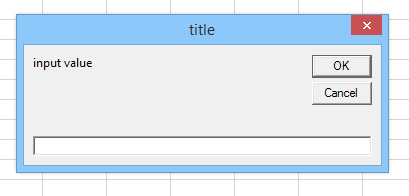This message box was create by creating in your macro the following Visual Basic code

Dim value As String
value = InputBox("Input Value", "Title")

This it is the simplest way to write it. Because it is an input form, you are looking to get a data from the user that you can then work with, in that case "value"

So you first declare it with the command DIM for Dimension and you write its type in that case it is a STRING (it must be a string).

The second line is the InputBox itself, with the following structure:

Input Box( Prompt, Title, Default value, Xposition, Yposition)

Prompt: this is the message you give to the user. It is a string

Title: this is the Title appearing at the top of the window

Default value: displays a default value in the inputbox field. Very useful in order to suggest a value.

Xposition and YPosition: if you want to locate it in the screen somewhere else than the center. The unit is Twips oo 1/20 of a point. So multiply your coordinate by 20.

Dim value As String
Dim Prompt As String
Dim Title As String

Prompt = "input value"
Title = "title"
value = InputBox(Prompt, Title)

this is the simplest form to write it

Let's display the input of the user on the Excel Table.

So first create a Button like it is explain in this button section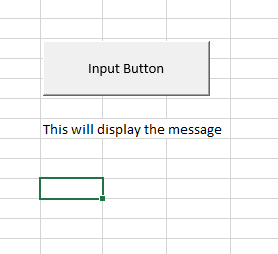By right clicking on the button, press assign macro then new (if you already have macro, then press EDIT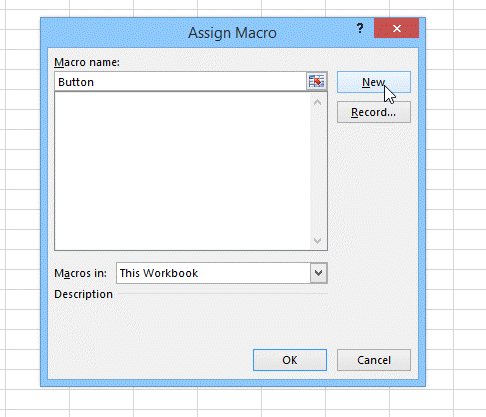The VBA editor will open and you can type the following code.

You can enter a value for X and Y but it is not necessary. The only necessary value is the Prompt

Sub myInputBox()

Dim DefaultResponse As String
Dim Xpos As Integer
Dim YPos As Integer
Dim value As String
Dim Prompt As String
Dim Title As String

Prompt = "input value"
Title = "title of my Input Box"
DefaultResponse = "if you don't chose something else, I decide for you"
value = InputBox(Prompt, Title, DefaultResponse)
' value = InputBox(Prompt, Title, DefaultResponse, Xposition, Yposition)
'I left X and Y empty so to have the message in the center of the screen

MsgBox (value)
'this display the value in a message box

Range("B10").value = value
'This display the message in cell B10

End Sub

This is what will be shown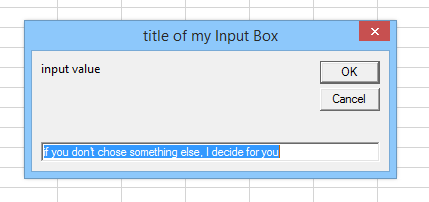Then the Message box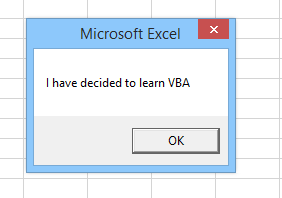Then the cell B10 is changed into the message you entered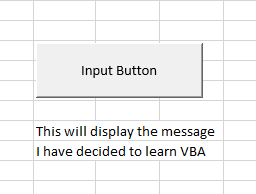Using VBA, Transform or convert the Input Box Text value into a Number

As the Inputbox returns a text or string, If you want to use it for calculation, you must turn it into a number first.

To do this use the expression:

Dim numbers As Integer
Dim inputtext As String
inputtext = InputBox("number of randoms")
numbers = Val(inputtext)
'the type of numbers is an integer that you can then use for calculation.

This should give you enough to start programming message boxes in VBA by yourself. Good Luck.